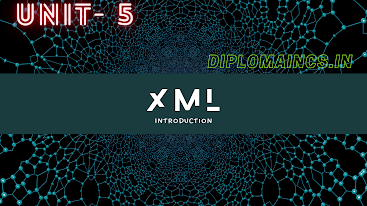## 12/27/20

### CPTC Question Lateral Entry 2020

#### Computer Programming Through "C" (Asked question for LE)

Sub Code: - 1600302

(Lateral Entry)

2019(ODD)

Time : 3Hrs

Semester  III(New)/CSE

CPTC

Full Marks : 70

Pass marks : 28

Group A

Choose the most suitable answer from the following options: -   (1*20=20)

(i) The file structure is defined in which of the following:-

(a) stdlib.h

(b) stdio.h

(c)  io.h

(d) conio.h

(ii) if int S is a one-dimension array of integers, which of the following refers to the third (3rd) element in the array.

(a) *(S+2)

(b) *(S+3)

(c) S+3

(d) S+2

(iii) An array is a collection of: -

(a) Different data types scattered throughout memory

(b) The same data types scattered throughout memory

(c) The same data type placed next to each other in memory

(d) Different data types placed next to each other in memory

(iv) What is the difference between the 5’s in these two expressions?

int num;                    num=11;

(a) First is particular element, second is type

(b)First is array size, second is particular element

(c) First is particular element, second is array size

(d) Both specify array size

(v) A preprocessor directive is: -

(a) A message from compiler to the programmer

(b) A message from programmer to the preprocessor

(c) A message from programmer to the microprocessor

(d)A message from compiler to linker

(vi) A header file is: -

(a) A file that contains standard library functions

(b) A file that contains definitions and macros

(c) A file that contains user defined functions

(d) A file that present in current working directory

(vii) All macro substitutions in a program are done: -

(a) Before compilation of the program

(b) After Compilation

(c) During Execution

(d)None of the above

(viii) In a program the statement:

#include “filename”

is replaced by the contents of the file “filename”

(a) Before compilation

(b) After Compilation

(c) During Compilation

(d) None of the above

(ix) A do-while loop is useful when we want that the statements within the loop must be executed

(a) Only once

(b) At least once

(c) More than once

(d) None of the above

(x) In what sequence the initialization, testing and execution of body is done in a do-while loop?

(a) Initialization, execution of body, testing

(b) Execution of body, initialization, testing

(c) Initialization, testing, execution of body

(d) None of the above

(xi) Which of the following are invalid C constants?

(a) 35;550

(b) 3.25e2

(c) 2 e^-3

(d) “show”

(xii) Which of the following are invalid variable name?

(a) int

(b) \$ hello

(c) number

(d) total Area

(xiii) “scanf()” is defined in which header file?

(a) conio.h

(b) stdio.h

(c) math.h

(d) graphics.h

(xiv)How many bits in one nibble?

(a) 16

(b) 8

(c) 4

(d) 2

(xv) ANSI Stands for:

(a)  American National Standards Institute

(b) Asian National Standards Institute

(c)  African National Standards Institute

(d) Arabian National Standards Institute

(xvi) “sqrt()” is defined in which header file?

(a)  conio.h

(b) stdio.h

(c)  math.h

(d) graphics.h

(xvii) What is the meaning of “&&” operator?

(a)  Logical AND

(b) Logical NOT

(c)  Logical OR

(d) None of the above

(xviii) How many keyword available in ‘C’?

(a)  16

(b) 32

(c)  64

(d) 128

(xix) ASCII stands for:

(a)  American Standard Code for Information Interchange

(b) Another Safe Code for Information Interchange

(c) All Sufficient Code for Information Interchange

(d) All of the above

(xx) Who is the developer of ‘C’ language?

(a)  C. Babhbage

(b) Hawkings

(c)  D. Ritchie

(d) T. Vaughan

Group:-"B"

Answer all Five Questions: -                         (5*4=20)

2.  How pointers differ from variables in C? Explain

OR

What is indirection operator and what is its role?

3.  Mention the advantages of structure type over union type with suitable example.

OR

What is the relationship between a member and a structure?

4. What is a computer program?

OR

What is a text editor?

5.  Write the algorithm and draw the flowchart to find the average of 5 numbers.

OR

What are the library functions? Mention any four library functions in C.

6. What is the purpose of scanf() function? How it is used within a C program?

OR

Explain the significance of the following control specifies

a.    %c

b.    %o

c.     %x

d.    %d

Group:- "C"

Answer all Five Questions: -                         (5*6=30)

7.  Write a C program that will read the value of x and evaluate the function

y (x) = x2+2x-10 if x<10

y(x) = |x| if x<0 using if statement

OR

Write a C program to find the roots of a quadratic equations ax2+bx+c=0 for all combinations of a, b and c using switch statement.

8.  Write a C program to accept an integer number between 1 and 9. Write the value of the number in words.

OR

Write a C program to print Armstrong numbers from 100 to 999.

9.  Write a C program to test whether a given number is a palindrome number. Explain the working of the program

OR

Write a C program to add m x n matrices and store the results in a third matrix.

10.  Define following C statements

a.          Break

b.          Continue

c.          Exit

OR

Define function. Explain prototype of function with suitable example.

11.  Explain various types of comments used in “C” language with suitable example.

OR

Write dawn the general syntax of switch statement and explain with help of example.